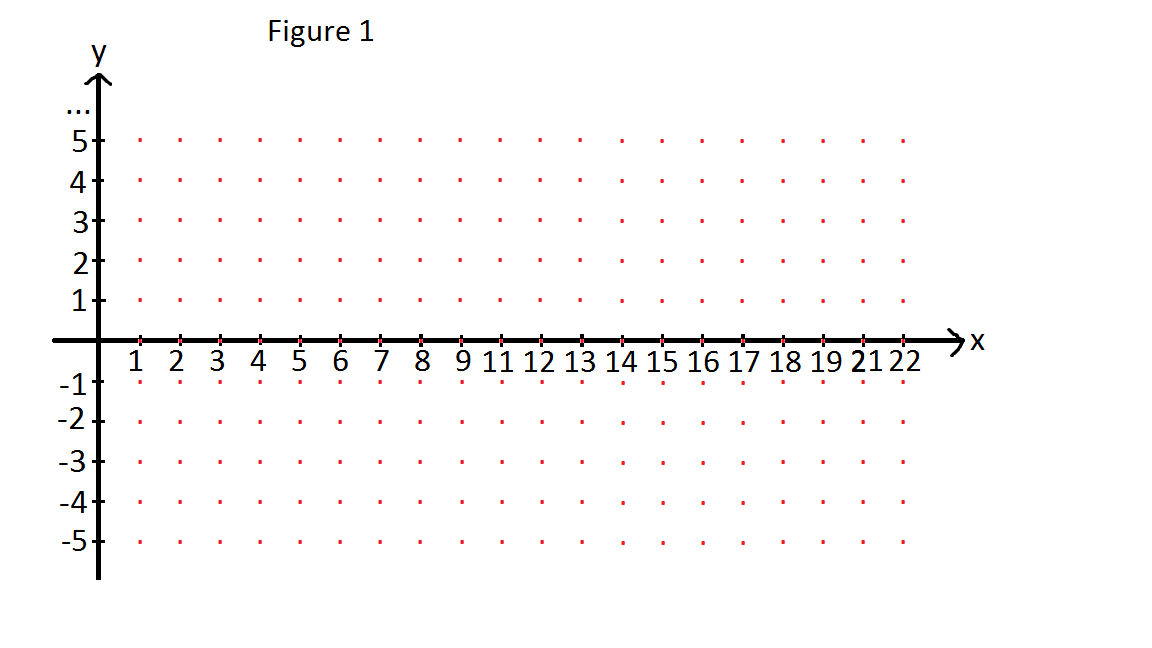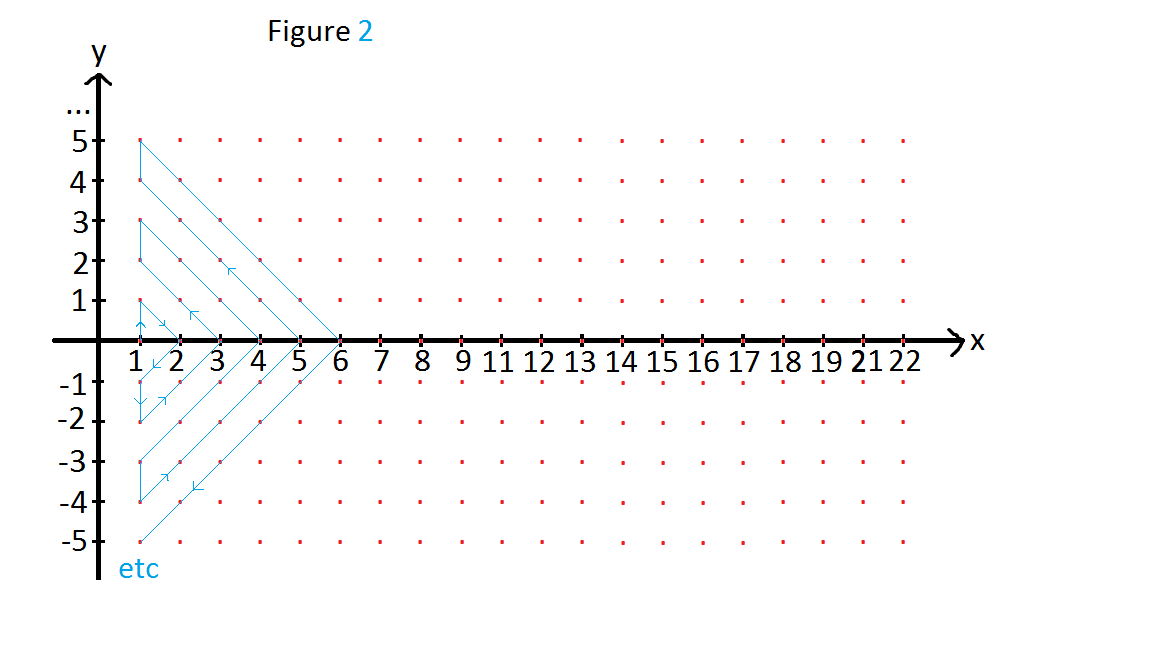# How to list all the elements of the set of real numbers?

I heard that the set of real numbers is uncountably infinite, meaning that it is not possible to list out all the real numbers in an orderly manner. I thought of a way to list out all the real numbers, in sequence. Can someone tell me if there is a mistake in it? Or is this method correct, and Cantor was wrong?

Step 1: draw a graph of y against x, where x is positive. Consider only all points (x,y) such that both x and y are integers. Omit all multiples of 10, for the x axis. This is so that numbers do not repeat. Refer to Figure 1.Step 2: The coordinates of (x, y) will represent the numbers that are in the set of real numbers. If the coordinates are (2, 4) then that point represents the number $2 \times 10^{4}$ In general, if the coordinates are (x,y) then the number that the point represents is $x \times 10^{y}$.

Step 3: We can then put the numbers in a sequence, as shown in Figure 2.We can continue the blue line pattern so that all points are covered, but it is not shown in the picture, because my computer does not have infinite space!

So the sequence is now,

1, 10, 2, 0.1, 0.01, 0.2, 3, 20, 100, 1000, 200, 30, 4 etc.

Step 4: Now we have to add in the negative numbers. We can slot the negative numbers between the positive numbers. We also have to add in 0, which can be put at the front.

So the sequence is now,

0, 1, -1, 10, -10, 2, -2, 0.1, -0.1, 0.01, -0.01, 0.2, -0.2, 3, -3, 20, -20, 100, -100, etc.

That's it! I have now listed every real number. Now to test this method.

Is 12345.6789 in the list?

Yes, its coordinates are at (123456789, -4). Thus, it is in the list because every coordinate if the graph is in the list.

Is $\frac {1}{3}$ in the list?

Let's first find 0.3. It is at (3,-1). 0.33 is at (33, -2). 0.333 is at (333, -3). In this way, $\frac {1}{3}$ would be at (333..., -$\infty$). Haha!

Is $\pi$ in the list?

Yes. It is at (3141592653..., -$\infty$)

I'm not sure if putting infinities as coordinates in a graph is acceptable, because

$\infty$-$\infty$ is undefined. However, the list is also infinitely long!Note by Lee Isaac
6 years, 2 months ago

This discussion board is a place to discuss our Daily Challenges and the math and science related to those challenges. Explanations are more than just a solution — they should explain the steps and thinking strategies that you used to obtain the solution. Comments should further the discussion of math and science.

When posting on Brilliant:

• Use the emojis to react to an explanation, whether you're congratulating a job well done , or just really confused .
• Ask specific questions about the challenge or the steps in somebody's explanation. Well-posed questions can add a lot to the discussion, but posting "I don't understand!" doesn't help anyone.
• Try to contribute something new to the discussion, whether it is an extension, generalization or other idea related to the challenge.

MarkdownAppears as
*italics* or _italics_ italics
**bold** or __bold__ bold
- bulleted- list
• bulleted
• list
1. numbered2. list
1. numbered
2. list
Note: you must add a full line of space before and after lists for them to show up correctly
paragraph 1paragraph 2

paragraph 1

paragraph 2

[example link](https://brilliant.org)example link
> This is a quote
This is a quote
    # I indented these lines
# 4 spaces, and now they show
# up as a code block.

print "hello world"
# I indented these lines
# 4 spaces, and now they show
# up as a code block.

print "hello world"
MathAppears as
Remember to wrap math in $$ ... $$ or $ ... $ to ensure proper formatting.
2 \times 3 $2 \times 3$
2^{34} $2^{34}$
a_{i-1} $a_{i-1}$
\frac{2}{3} $\frac{2}{3}$
\sqrt{2} $\sqrt{2}$
\sum_{i=1}^3 $\sum_{i=1}^3$
\sin \theta $\sin \theta$
\boxed{123} $\boxed{123}$